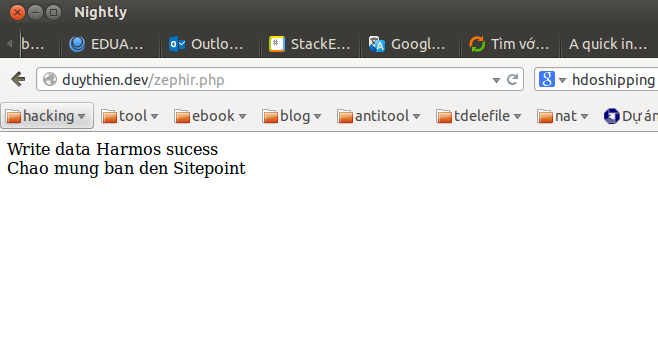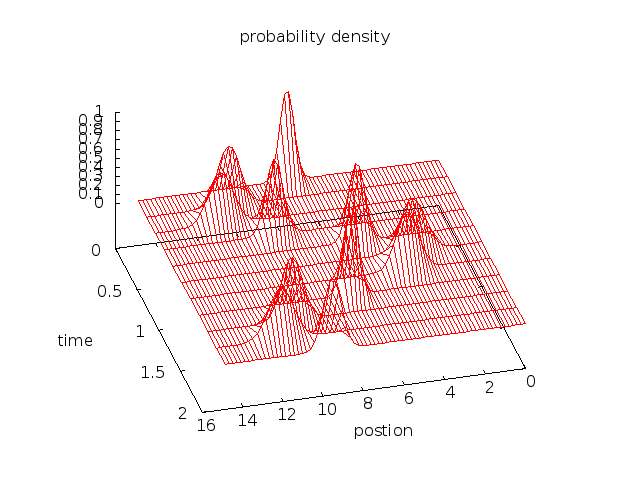# Getting Started with PHP Extension Development via Zephir

PHP

Share:

#### Free JavaScript Book!Write powerful, clean and maintainable JavaScript.

RRP \$11.95

This tutorial will explain how to create a PHP extension using a new language: Zephir, which is similar to C and Fortran. You can download the full source code from github. We’ve touched on the concept of Zephir before, so if you’re interested in getting a broad overview, see our previous articles.

Zephir can be looked at as a hybrid language that lets you write code that looks like PHP, but is then compiled to native C, meaning you can create an extension from it and come away with very efficient code.

## Installation

To build a PHP extension and use Zephir you’ll need the following:

• gcc >= 4.x/clang >= 3.x/vc++ 9
• gnu make 3.81 or later
• php development headers and tools
• re2c 0.13 or later
• json-c

The installation instructions vary for every platform, so I trust you’ll know how to obtain them if you’re reading an article with a topic as advanced as this one. For the record – I recommend using a Linux based system for developing Zephir apps.

``````git clone https://github.com/phalcon/zephir
cd zephir && ./install -c``````

It should install automatically – try typing `zephir help`. If it is not working, add the “bin” directory to your PATH enviroment variable. In my case: `/home/duythien/app/zephir/bin`, like so:

``````vi \$HOME/.bash_profile
``````

Append the following export command:

``````export PATH=\$PATH:/home/duythien/app/zephir/bin
``````

To verify the new path settings and test the installation, enter:

``````echo \$PATH
zephir help
``````

You can find out about Zephir basics and syntax, as well as its typing system and see some demo scripts over at their website.

### Programming with Zephir

Now we’ll use Zephir to re-work a mathematical equation that C and Fortran handle very well. The example is rather esoteric and won’t be explained into much detail, except to demonstrate the power of Zephir.

#### Time-Dependent Schrodinger Equation solved with Finite Difference

The time-dependent Schrödinger equation can be solved with both implicit (large matrix) and explicit (leapfrog) methods. I’ll use the explicit method.

Firstly, issue the following command to create the extension’s skeleton:

``````zephir init myapp
``````

When this command completes, a directory called “myapp” is created on the current working directory. This looks like:

``````myapp/
|-----ext/
|-----myapp/
|-----config.json
``````

Inside the “myapp” folder, create a file called “quantum.zep” (which will give us the `Myapp\Quantum` namespace). Copy paste the following code inside:

``````namespace Myapp;
class Quantum{

const PI = 3.14159265358979323846;
const MAX = 751;

public function Harmos(double x){
int  i,j,n;
var  psr, psi, p2, v,paramater,fp;
double dt,dx,k0,item_psr,item_psi;

let dx = 0.02,
k0 = 3.0*Myapp\Quantum::PI,
dt = dx*dx/4.0;
let paramater =[dx,k0,dt,x];
let i   = 0,
psr = [],
psi = [],
p2  = [],
v   = [],
fp  = [];

let fp = fopen ("harmos.txt", "w");
if (!fp) {
return false;
}
while i <= Myapp\Quantum::MAX{
let item_psi = sin(k0*x) / exp(x*x*2.0),
item_psr = cos(k0*x) / exp(x*x*2.0);
let psr[i] = [item_psr],
psi[i] = [item_psi],
v[i] = [5.0*x*x],
x = x + dx,
i++;
}
var tmp;
let i =1, j=1,tmp=[2.0];
for n in range(0, 20000){

for i in range(1,Myapp\Quantum::MAX - 1 ){
let psr[i] =psr[i] - paramater*(psi[i+1] + psi[i - 1]
- tmp*psi[i]) / (paramater*paramater) + paramater*v[i]*psi[i],

p2[i] = psr[i]*psr[i] + psi[i]*psi[i];
}
for j in range(1,Myapp\Quantum::MAX - 1 ) {
let psr = 0,
psr[Myapp\Quantum::MAX]= 0 ;
let psi[j] = psi[j] + paramater*(psr[j+1] + psr[j - 1]
- tmp*psr[j]) / (paramater*paramater) - paramater*v[j]*psr[j];
}
//output split
if (n ==0 || n % 2000 == 0) {
let i =1;
while i < Myapp\Quantum::MAX - 1 {
fprintf(fp, "%16.8lf %16.8lf %16.8lf \n",i*dx,n*dt,p2[i]);
let i = i + 10;
}
fprintf(fp, "\n");
}
// change new->old
let j = 1;
while j <  Myapp\Quantum::MAX - 1 {
let psi[j] = psi[j],
psr[j] = psr[j];
let j++;
}

}
return true;

}
}
``````

We’ve used many PHP functions such as fopen(), sin(), fprintf(), etc – feel free to study the syntax. I’ll also give you one more example. In the process of working with the Phalcon PHP framework, the function Phalcon\Tag::friendlyTitle() is invalid if you’re working in Vietnamese or German. This example, far simpler than the equation above, creates the file `normalizeChars.zep`. Insert the following code into the file:

``````namespace Myapp;

class NormalizeChars{
public function trans(var s)
{
var replace;
let replace = [
"ế" : "e",
"ề" : "e",
"ể" : "e",
"ễ" : "e",
"ệ" : "e",
//--------------------------------E^
"Ế" : "e",
"Ề" : "e",
"Ể" : "e",
"Ễ" : "e",
"Ệ" : "e",
//--------------------------------e
"é" : "e",
"è" : "e",
"ẻ" : "e",
"ẽ" : "e",
"ẹ" : "e",
"ê" : "e",
//--------------------------------E
"É" : "e",
"È" : "e",
"Ẻ" : "e",
"Ẽ" : "e",
"Ẹ" : "e",
"Ê" : "e",
//--------------------------------i
"í" : "i",
"ì" : "i",
"ỉ"  : "i",
"ĩ" : "i",
"ị" : "i",
//--------------------------------I
"Í" : "i",
"Ì" : "i",
"Ỉ"  : "i",
"Ĩ" : "i",
"Ị" : "i",
//--------------------------------o^
"ố" : "o",
"ồ" : "o",
"ổ" : "o",
"ỗ" : "o",
"ộ" : "o",
//--------------------------------O^
"Ố"  : "o",
"Ồ" : "o",
"Ổ"  : "o",
"Ô" : "o",
"Ộ"  : "o",
//--------------------------------o*
"ớ"  : "o",
"ờ" : "o",
"ở"  : "o",
"ỡ" : "o",
"ợ"  : "o",
//--------------------------------O*
"Ớ"  : "o",
"Ờ" : "o",
"Ở"  : "o",
"Ỡ" : "o",
"Ợ"  : "o",
//--------------------------------u*
"ứ"  : "u",
"ừ" : "u",
"ử"  : "u",
"ữ" : "u",
"ự"  : "u",
//--------------------------------U*
"Ứ"  : "u",
"Ừ" : "u",
"Ử"  : "u",
"Ữ" : "u",
"Ự"  : "u",
//--------------------------------y
"ý"  : "y",
"ỳ" : "y",
"ỷ"  : "y",
"ỹ" : "y",
"ỵ"  : "y",
//--------------------------------Y
"Ý"  : "y",
"Ỳ" : "y",
"Ỷ"  : "y",
"Ỹ" : "y",
"Ỵ"  : "y",
//--------------------------------DD
"Đ"  : "d",
"đ" : "d",
//--------------------------------o
"ó"  : "o",
"ò" : "o",
"ỏ"  : "o",
"õ" : "o",
"ọ"  : "o",
"ô" : "o",
"ơ"  : "o",
//--------------------------------O
"Ó"  : "o",
"Ò" : "o",
"Ỏ"  : "o",
"Õ" : "o",
"Ọ"  : "o",
"Ô" : "o",
"Ơ"  : "o",
//--------------------------------u
"ú"  : "u",
"ù" : "u",
"ủ"  : "u",
"ũ" : "u",
"ụ"  : "u",
"ư" : "u",
//--------------------------------U
"Ú"  : "u",
"Ù" : "u",
"Ủ"  : "u",
"Ũ" : "u",
"Ụ"  : "u",
"Ư" : "u",

//--------------------------------a^
"ấ"  : "a",
"ầ" : "a",
"ẩ"  : "a",
"ẫ" : "a",
"ậ"  : "a",
//--------------------------------A^
"Ấ"  : "a",
"Ầ" : "a",
"Ẩ"  : "a",
"Ẫ" : "a",
"Ậ"  : "a",
//--------------------------------a(
"ắ"  : "a",
"ằ" : "a",
"ẳ"  : "a",
"ẵ" : "a",
"ặ"  : "a",
//--------------------------------A(
"Ắ"  : "a",
"Ằ" : "a",
"Ẳ"  : "a",
"Ẵ" : "a",
"Ặ"  : "a",
//--------------------------------A
"Á"  : "a",
"À" : "a",
"Ả"  : "a",
"Ã" : "a",
"Ạ"  : "a",
"Â" : "a",
"Ă"  : "a",
//--------------------------------a
"ả"  : "a",
"ã" : "a",
"ạ"  : "a",
"â" : "a",
"ă"  : "a",
"à" : "a",
"á"  : "a"];
return strtr(s, replace);

}
}
``````

Now, we need to tell Zephir that our project must be compiled and the extension generated:

``````cd myapp
zephir build
``````

On the first time it is run a number of internal commands are executed producing the necessary code and configurations to export this class to the PHP extension. If everything goes well you will see the following message at the end of the output:

Compiling…
Installing…
Extension installed!
Don’t forget to restart your web server

Note that since Zephir is will in its infancy, it’s possible to run into bugs and problems. The first time I tried to compile this it didn’t work. I tried the following commands and eventually got it to work:

``````zephir compile
cd ext/
phpize
./configure
make && sudo make install
``````

The last command will install the module in the PHP extensions folder (in my case: `/usr/lib/php5/20121212/`). The final step is to add this extension to your php.ini by adding the following line:

``````extension=/usr/lib/php5/20121212/myapp.so
//or
extension=myapp.so
``````

Restart Apache, and we’re done.

#### Test the code

Now, create a new file called zephir.php :

``````\$flow = new Myapp\Quantum();

\$ok = \$flow->Harmos(-7.5);

if (\$ok == true) {
echo "Write data Harmos sucess <br>";
}

\$normalize = new Myapp\NormalizeChars();

echo \$normalize->trans("Chào mừng bạn đến Sitepoint");
``````

Finish up by visiting your `zephir.php` page. It should look similar to the following output:If you’re mathematically inclinced, install gnuplot and run it with the .txt output we got from our Zephir extension:

``````gnuplot
splot './harmos.txt' w l
``````

This command will draw the image using the data file harmos.txt, which will look like this, proving our equation was calculated properly.### Protected code

In some cases, the compilation does not significantly improve performance, maybe because of a bottleneck located in the I/O bound of the application (quite likely) rather than due to limits in computation or memory. However, compiling code could also bring some level of intellectual protection to your application. When producing native binaries with Zephir, you can also hide the code from users or customers – Zephir allows you to write closed source PHP applications.

### Conclusion

This article gave a basic guide on how to create extensions in Zephir. Remember, Zephir wasn’t created to replace PHP or C, but as a complement to them, allowing developers to venture into code compilation and static typing. Zephir is an attempt to join the best things from the C and PHP worlds and make applications run faster, and as such competes rather directly with HHVM and Hack.### Thien Tran Duy

I'm a professional web developer from Vietnam with B.S Physics and Engineering. I'm a Phalcon enthusiast as Opensource. In free time, I was support on forum Phalcon also my forum and contribution core Phalcon.

#### New books out now!